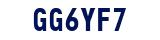# CBSE Sample Papers class 8 Maths

The following Sample papers are prepared chapter wise. Each chapter consist of questions based on CBSE Syllabus. Our academic team uploaded quality questions with detail solutions of each and every question. CBSE Sample Papers class 8 Maths must be solve after reading the theory of recommended text book.### How CBSE Sample Papers Class 8 Maths can help you to build concepts

Maths is subject of practice. The best way to practice class 8 maths is solving questions. To help you in this Academic team of Physics Wallah uploaded CBSE Sample Papers class 8 Maths.Each Sample paper consist of solved and unsolved questions with solutions.

For additional information related to the subject you can check the Maths Formula and Maths Doubts section.

### Why one must solve CBSE Sample Papers Class 8 Maths

To become expert in any Maths students must focus on solving questions. To do effective practice you need quality of questions in Maths and solve all questions by yourself for more effectiveness try to solve each sheet in the given period of time.

### How to use Class 8 Maths CBSE Sample Papers

CBSE Sample Papers class 8 Maths must be use after proper revision of your lesion. Start form first chapter read it thoroughly from your text book try to revise your notes. Once you are confident that you know and understand the chapter start solving the CBSE Sample Papers class 8 Maths . Do solve questions from NCERT exercise with the help of NCERT Solutions for Class 8 Maths prepared by Physics Wallah.

Physics Wallah uploaded lot of resource for class 8 Maths apart from worksheet. Like for class 8 Maths you can download Important questions for class 8 Maths, reference book solution for class 8 Maths , Maths formulas sheet, online test for class 8 Maths, detail and in-depth theory for JEE and NEET aspirants for class 8 Maths.

Students can also access the RS Aggarwal Class 8 Maths Solutions from here.

Q1. Why CBSE class 8 maths sample papers are important?

Ans. The mathematical class 8 CBSE sample papers are a perfect test for students to verify the exam preparation. They could see where they are making mistakes and so they can work on those areas. This will improve their overall performance. Practicing sample papers is an effective way to get rid of exam anxiety. It gives students a sense of confidence and frees them from all worries on the day of the exam.

Q2. What are the benefits of solving CBSE class 8 maths sample papers?

Ans. When students solve CBSE sample papers, they learn how well prepared they are for the math exam and self-evaluate themselves by solving the latest CBSE sample papers.

• They will speed up their question-solving speed so they can solve all the questions during the exam.
• Solving questions over and over again puts all the important formulas at your fingertips. This will save time on the exam and resolve the paper quickly.
• By solving the class 8 sample papers students can revise all the major sections in a quick sequence of time.

Q3. What are CBSE class 8 maths sample papers?

Ans. CBSE class 8 sample papers are the craved material for exam purposes which makes students neighborly with actual exam paper. In class 8 weightage of the question, time management, exam pattern, and preparation strategy play a key role. Repeated practice of sample papers will boost your confidence and reduce your stress level in exams.

Q4. Does Physics Wallah provide solutions for the CBSE sample papers for class 8 maths?

Ans. We at Physics Wallah offer you class 8 maths sample mock papers. You can access it through our website or mobile app in PDF format for easy download. Another advantage is that they are free. All of these solutions have been prepared by our academic excellent subject matter experts, so you can easily introduce to them without a doubt.

Q5. How many sample papers should be practiced for CBSE class 8 maths?

Ans. It depends on whether your preparation is good enough and you have completed the entire class 8 maths syllabus and if you are confident enough in almost the topics that you don't have to solve many sample papers. But if some topics are good and you are unsure of the others, you need to solve 7-9 articles so that you can build confidence for the exam.

Q6. Which is the best website for class 8 maths sample papers?

Ans. We will only say that quality speaks, Students can find CBSE class 8 maths sample papers in PDF format. At Physics Wallah, we provide sample documents for class 8 maths with solutions from our experienced academic teachers. Class 8 maths sample papers students can download from our website.